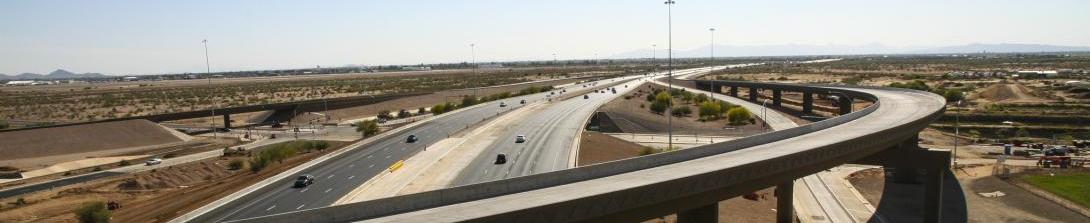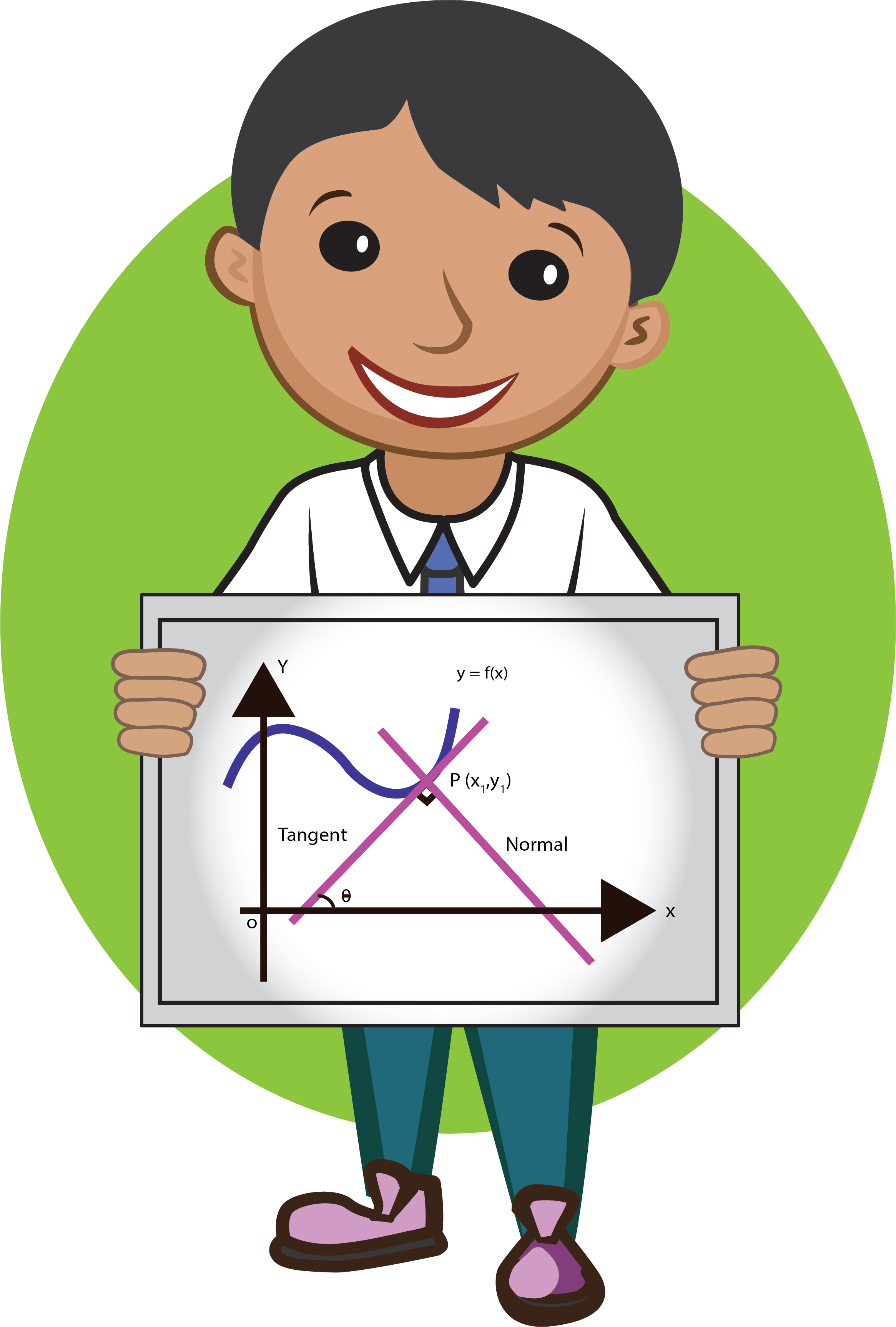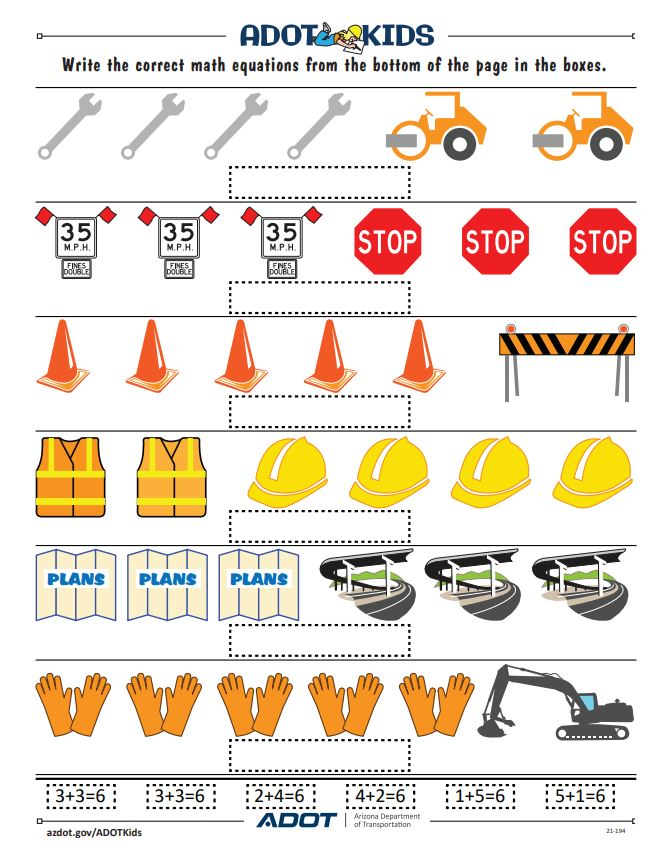# Civil Engineers use math to design, build and maintain transportation structures

## Civil Engineers use math to design, build and maintain transportation structures

By Audrey St. Clair / ADOT Communications
August 3, 2021Did you know there are 26 different types or branches of math? Math is not just solving number problems — it is also needed for physics, chemistry and engineering! Civil engineers, like the ones at ADOT, use nearly every form of math at one point in time to do their job. It’s important to have a good foundation of math, especially if you are interested in a career as an engineer. As you progress through school, you can learn more advanced types of math. Physics and chemistry are also important for civil engineers to learn. Civil engineers do not spend all of their time on math, but when it’s needed, they have to be very comfortable with the different branches of math, especially those that deal with physics.

Here is an overview of the types of math and how civil engineers use them in their work:

• Algebra is used by civil engineers on a daily basis. It is a branch of mathematics in which letters and other symbols are used to represent numbers and quantities, and is used to solve equations and find statistics.
• Calculus is defined as the mathematical study of change in respect to time, heat, wave, electric current, vibrations and the relationships of the different parts of the problem. An example of calculus in action is the relationship between a car’s speedometer (measures speed) and the odometer (measures distance traveled). One, if unknown (called a variable), can be calculated using the other.
• Civil engineers use trigonometry when surveying a structure. Surveying deals with land elevations as well as the various angles of structures. Trigonometry is the study of the sides and angles, and their relationship in triangles.
•Geometry is used to design with the best angles to make structures as strong as possible, using shape, size, position and other properties. Civil engineers use geometry to design and assemble shapes to construct freeways, tunnels, bridges and more.

Civil engineers use math equations that are derived from chemistry. Chemistry’s equations are used to measure the strength of materials. Engineers use these equations to select the right material for a project, for example, to determine the amount of force a beam can handle and to figure out where to place supports on a bridge.

Civil engineers also use physics. When a bridge is being designed, physics is used to figure out how large the supporting piers should be, how thick the steel columns of the bridge need to be and how many of them should be installed. Physics equations typically use a combination of algebra, calculus and trigonometry.

Math is a really important part of a civil engineer’s job. At places like ADOT, the planning phase of the project (design) and the budget (how much it will cost) are all based upon mathematical calculations by the engineers. These calculations are also used to ensure safety and durability of the project by using the right measurements and materials.